# JEE Main 2020 Maths Paper With Solutions Shift 2 Sept 3

JEE Main 2020 Maths paper September 3 Shift 2 solutions are available on this page. Students are advised to revise and learn these solutions, so that they can score higher ranks for the JEE Main exam. These solutions are given in a step by step method to help students easily understand the problems. You can easily download the PDF format of these solutions for free

### September 3 Shift 2 - Maths

1. If x3dy+xy dx = x2dy+2y dx; y(2) = e and x>1, then y(4) is equal to:

1. a) √e/2
2. b) (3/2)√e
3. c) (1/2)+√e
4. d) (3/2)+√e

Solution:

1. (x3-x2)dy = (2-x) ydx

$$\int \frac{dy}{y}=\int \frac{2-x}{x^{2}(x-1)}dx$$

$$\int \frac{dy}{y}=-\int \frac{x-2}{x^{2}(x-1)}dx$$

$$\int \frac{dy}{y}=-\int (\frac{p}{x}+\frac{q}{x^{2}}+\frac{r}{x-1})dx$$

p = 1, q = 2, r = -1

$$\int \frac{dy}{y}=\int \frac{-1}{x}dx-\int\frac{2}{x^{2}}dx-\int\frac{-1}{x-1}dx$$

ln|y| = -ln x+(2/x)+ln (x-1)+c

x = 2, y = e

1 = 1-ln 2+c

⇒ c = ln 2

ln|y| = (2/x)-ln |x|+ln |x-1|ln 2

put x = 4

ln|y| = (1/2)-2ln 2+ ln 3+ ln 2

ln y = ln(3/2)+(1/2)

y = (3/2)×e1/2

= (3/2)√e

2. Let A be a 3×3 matrix such that adj A =

$$\begin{bmatrix} 2 & -1 &1 \\ -1 &0 &2 \\ 1 & -2 & -1 \end{bmatrix}$$
and B = adj(adj A) . If |A| = λ and |(B-1)T| = μ, then the ordered pair, (|λ|,μ) is equal to:

1. a) (9, 1/81)
2. b) (9, 1/9)
3. c) (3, 1/81)
4. d) (3, 81)

Solution:

$$\begin{bmatrix} 2 & -1 &1 \\ -1 &0 &2 \\ 1 & -2 & -1 \end{bmatrix}$$

⇒ |A2| = 9

⇒ |A| = 3 = |λ| and |A| = -3 = |λ|

|B| = ((|A|)(3-1))2 = |A|4 = 34 = 81

|(BT)-1| = 1/|(BT)|

= 1/|B|= 1/81=μ

Hence |λ|,μ = (3, 1/81)

3. Let a, b, cεR be such that a2+b2+c2 = 1, if a cos θ = b cos(θ+2π/3) = c cos(θ+4π/3), where θ = π/9, then the angle between the vectors

$$a\hat{i}+b\hat{j}+c\hat{k}$$
and
$$b\hat{i}+c\hat{j}+a\hat{k}$$
is:

1. a) π/2
2. b) 2π/3
3. c) π/9
4. d) 0

Solution:

1. cos α =

$$\frac{\vec{p}.\vec{q}}{\left | p \right |\left | q \right |}$$

= (ab+bc+ca)/a2+b2+c2

= (ab+bc+ca)/1

cos α = ab+bc+ca

cos α = abc[(1/a)+(1/b)+(1/c)] ..(i)

a cos200 = b cos(1400) = c cos(2600) = λ

⇒ (1/a) = cos 200

(1/b) = cos 1400

(1/c) = cos 2600

Put in (i)

cos α = (abc/λ)(cos 200+ cos 1400+ cos 2600)

cos α = (abc/λ)(cos 200+ 2cos 2000+ cos 600)

cos α = (abc/λ)(cos 200- cos 200)

cos α = 0

α = π/2

4. Suppose f(x) is a polynomial of degree four, having critical points at (-1,0,1). If T = {xεR f(x) = f(0) }, then the sum of squares of all the elements of T is:

1. a) 6
2. b) 2
3. c) 8
4. d) 4

Solution:

1. f’(x) = k(x+1)x(x-1)

f’(x) = k[ x3-x]

Integrating both sides

f(x) = k[(x4/4)- (x2/2)]+c

f(0) = c

f(x) = f(0) ⇒ k[(x4/4)- (x2/2)]+c = c

⇒ k(x2/4)(x2-2) = 0

⇒ x = 0, √2, -√2

Sum of squares of all elements = 02+(√2)2+(-√2)2

= 4

5. If the value of the integral

$$\int_{0}^{\frac{1}{2}}\frac{x^{2}}{(1-x^{2})^{\frac{3}{2}}}dx$$
is k/6, then k is equal to:

1. a) 2√3+π
2. b) 3√2+π
3. c) 3√2-π
4. d) 2√3-π

Solution:

1. $$\int_{0}^{\frac{1}{2}}\frac{x^{2}}{(1-x^{2})^{\frac{3}{2}}}dx$$

Put x = sinθ

$$\int_{0}^{\frac{\pi }{6}}\frac{\sin ^{2}\theta }{\cos ^{3}\theta }\cos \theta .d\theta$$

=

$$\int_{0}^{\frac{\pi }{6}}\tan ^{2} \theta .d\theta = [\tan \theta -\theta]_{0}^{\frac{\pi }{6}}$$

⇒ (1/√3)-(π/6) = k/6

(2√3-π)/6 = k/6

k = 2√3-π

6. If the term independent of x in the expansion of ((3/2)x2 -(1/3x))9 is k, then 18 k is equal to:

1. a) 5
2. b) 9
3. c) 7
4. d) 11

Solution:

1. Tr+1 =

$$^{^{9}}C_{r}\left ( \frac{3}{2}x^{2} \right )^{9-r}\left ( \frac{-1}{3x} \right )^{r}$$

=

$$^{^{9}}C_{r}\frac{3^{9-r}}{2^{9-r}}(\frac{-1}{3})^{r}x^{18-3r}$$

18-3r = 0

⇒ r = 6

=

$$^{^{9}}C_{r}(\frac{3^{-3}}{2^{3}})=k$$

⇒ 7/18 = k

⇒ 18k = 7

7. If a triangle ABC has vertices A(-1,7), B(-7,1) and C(5,-5), then its orthocentre has coordinates;

1. a) (-3,3)
2. b) (-3/5, 3/5)
3. c) (3/5, -3/5)
4. d) (3,-3)

Solution:

1.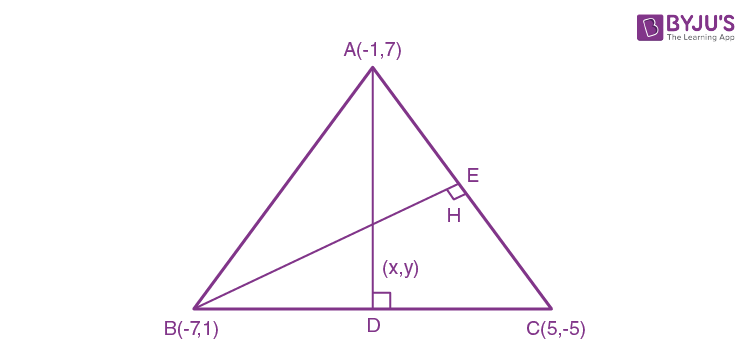mAH. mBC = -1

⇒ ((y-7)/(x+1))(1+5)/(-7-5) = -1

⇒ 2x-y+9 = 0 …..(i)

and mBH. mAC = -1

((y-1)/(x+7))(7--5)/(-1-5) = -1

x-2y+9 = 0 .....(ii)

Solving equation (i) and (ii) we get

(x, y) = (-3,3)

8. Let e1 and e2 be the eccentricities of the ellipse, (x2/25)+(y2/b2) = 1 (b<5) and the hyperbola, (x2/16)-(y2/b2) = 1 respectively satisfying e1e2 = 1. If α and β are the distances between the foci of the ellipse and the foci of the hyperbola respectively, then the ordered pair (α,β) is equal to:

1. a) (8,12)
2. b) (24/5,10)
3. c) (20/3,12)
4. d) (8,10)

Solution:

1. α = 10e1

b2 = 25(1-e12)

β = 8e2

b2 = 16(e22-1)

(e1e2)2 = 1

(1-b2/25)( 1+b2/16) = 1

⇒ 1+(b2/16)-(b2/25)-(b4/400) = 1

⇒ (9b2/16×25)-(b4/400) = 0

⇒ 9b2-b4 = 0

⇒ b2(9-b2) = 0, b ≠ 0

Therefore, 9-b2 = 0

b2 = 9

e1 = 4/5

α = 2ae1

= 10×4/5

= 8

e2 = 5/4

β = 2ae2

= 8×5/4

= 10

(α, β) = (8, 10)

9. If z1, z2 are complex numbers such that Re(z1) = |z1-1|, Re(z2) = |z2-1| and arg (z1-z2) = π/6, then Im(z1+z2) is equal to:

1. a) 2√3
2. b) 2/√3
3. c) 1/√3
4. d) √3/2

Solution:

1. z1 = x1+iy1

z2 = x2+iy2

x12 = (x1-1)2+y12

⇒ y12-2x1+1 = 0 ..(i)

x22 = (x2-1)2+y22

⇒ y22-2x2+1 = 0 ..(ii)

From equation (ii)-(i)

(y12-y22)+2(x2-x1) = 0

(y1+y2)(y1-y2) = 2(x1-x2)

(y1+y2) = 2(x1-x2)/ (y1-y2)

arg (z1-z2) = π/6

tan-1(y1-y2)/(x1-x2) = π/6

⇒ (y1-y2)/(x1-x2) = 1/√3

So y1+y2 = 2√3

10. The set of all real values of λ for which the quadratic equations, (λ2+1)x2-4λx+2 = 0 always have exactly one root in the interval (0,1) is:

1. a) (-3,-1)
2. b) (2,4)
3. c) (1,3)
4. d) (0,2)

Solution:

1. f(0) f(1) ≤ 0

⇒ (2) [λ2-4λ +3] ≤0

(λ-1)(λ- 3) ≤0

⇒ λ ε [1, 3]

at λ = 1

2x2 -4x+2 = 0

⇒ (x-1)2 = 0

x = 1, 1

⇒λ ε (1, 3]

11. Let the latus rectum of the parabola y2 = 4x be the common chord to the circles C1 and C2 each of them having radius 2√5. Then, the distance between the centres of the circles C1 and C2 is:

1. a) 8
2. b) 8√5
3. c) 4√5
4. d) 12

Solution:

1.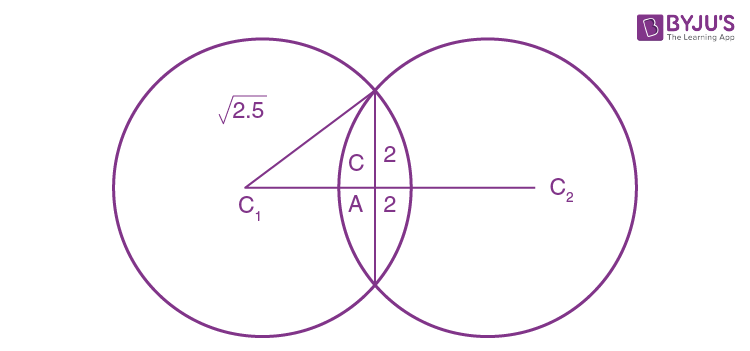C1C2 = 2C1A

(C1A)2 + 4 = ( 2√5)2

C1A = 4

C1C2 = 8

12. The plane which bisects the line joining the points (4,-2,3) and (2,4,-1) at right angles also passes through the point:

1. a) (0,-1,1)
2. b) (4,0,1)
3. c) (4,0,-1)
4. d) (0,1,-1)

Solution:

1.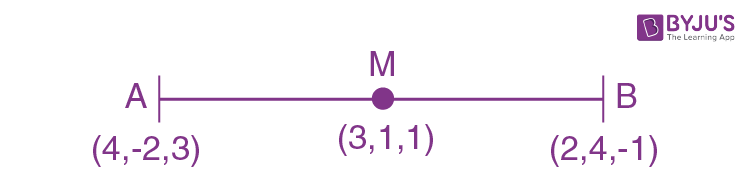a = 2, b = -6

c = 4

Equation of plane is

2(x-3)+(-6)(y -1)+ 4(z-1) = 0

⇒ 2x-6y+4z = 4 passes through (4, 0, -1).

13.

$$\lim_{x\to a}\frac{(a+2x)^{\frac{1}{3}}-(3x)^{\frac{1}{3}}}{(3a+x)^{\frac{1}{3}}-(4x)^{\frac{1}{3}}}$$
is equal to:

1. a) (2/9)4/3
2. b) (2/3)4/3
3. c) (2/3)(2/9)1/3
4. d) (2/9)(2/3)1/3

Solution:

1. Apply L hospital rule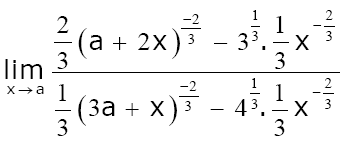⇒ [(2/3)(3a)-2/3-1/32/3(a-2/3)]/[(1/3)(4a)-2/3-(1/3)41/3a-2/3]

= (2/3)×(2/9)1/3

14. Let xi (1≤ i ≤10)be ten observations of a random variable X. If

$$\sum_{i=1}^{10}(x_{i}-p)=3$$
and
$$\sum_{i=1}^{10}(x_{i}-p)^{2}=9$$

where 0 ≠ p εR , then the standard deviation of these observations is :

1. a) 7/10
2. b) 9/10
3. c) √(3/5)
4. d) 4/5

Solution:

1. Standard deviation is free from shifting of origin

S.D = √variance

= √[(9/10)-(3/10)2]

= √[(9/10)-(9/100)]

= √(81/100)

= 9/10

15. The probability that a randomly chosen 5 digit number is made from exactly two digits

is :

1. a) 134/104
2. b) 121/104
3. c) 135/104
4. d) 50/104

Solution:

1. Total case = 9(104)

First case : Choose two non-zero digits = 9C2

Now, number of 5-digit numbers containing both digits = 25-2

Second case : Choose one non-zero and one zero as digit = 9C1

Number of 5-digit numbers containing one non-zero and one zero both = 24-1.

fav. case = 9C2(25-2)+ 9C1(24-1)

= 1080+135

= 1215

Probability = 1215/9×104

= 135/104

16. If

$$\int \sin ^{-1}\left ( \sqrt{\frac{x}{1+x}} \right )dx= A(x)\tan ^{-1}(\sqrt{x})+B(x)+C$$
, where C is a constant of integration, then the ordered pair (A(x), B(x)) can be:

1. a) (x+1, -√x)
2. b) (x-1, -√x)
3. c) (x+1, √x)
4. d) (x-1, √x)

Solution:

1.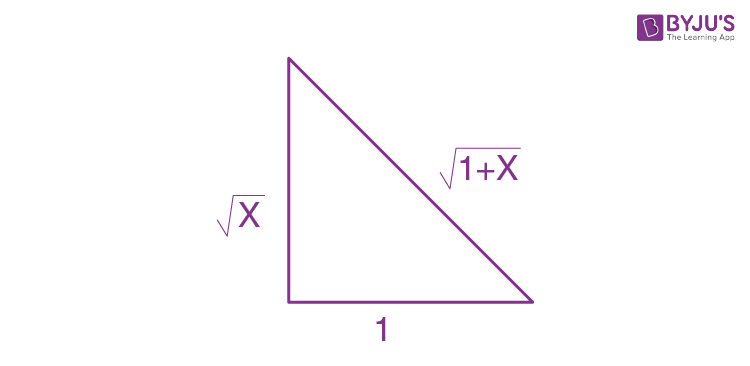Given

$$\int \sin ^{-1}\left ( \sqrt{\frac{x}{1+x}} \right )dx= A(x)\tan ^{-1}(\sqrt{x})+B(x)+C$$

∫tan-1√x. 1 dx

Here tan-1√x is the first function and 1 dx is the second function.

Integrating we get

(tan-1√x)x-∫(x/1+x)×(1/2√x)dx

Put x = t2

dx = 2t dt

(tan-1√x)x-∫(x/1+x)×(1/2√x)dx = x(tan-1√x)- ∫(t2)(2tdt)/(1+t2)2t

= x(tan-1√x)-t+tan-1t+c

= x(tan-1√x)-√x+tan-1√x+c

= tan-1√x(x+1)-√x+c

A(x) = x+1

B(x) = -√x

17. If the sum of the series

$$20+19\frac{3}{5}+19\frac{1}{5}+18\frac{4}{5}+...$$
upto nth term is 488 and the nth term is negative, then:

1. a) n = 60
2. b) n = 41
3. c) nth term is -4
4. d) nth term is
$$-4\frac{2}{5}$$

Solution:

1. $$20+19\frac{3}{5}+19\frac{1}{5}+18\frac{4}{5}+...$$

Sn = 488

⇒ (n/2)(2×20+(n-1)(-2/5)) = 488

⇒ 20n-(n2/5)+(n/5) = 488

⇒ 100n-n2+n = 2440

⇒ n2-101n+2440 = 0

⇒ n = 61 or 40

For n = 40, Tn = 20+39(-2/5) = +ve

n = 61, Tn = 20+60(-2/5) =20-24 = -4

18. Let p, q, r be three statements such that the truth value of (p˄q) -> (∼q˅r) is F. Then the truth values of p, q, r are respectively:

1. a) F, T, F
2. b) T, F, T
3. c) T, T, F
4. d) T, T, T

Solution:

1. (p˄q) -> (∼q˅r) possible when p˄q -> T

∼q˅r -> F

p -> T ; p˄q⇒ T

q -> T ; ∼q˅r -> F˅F ⇒ F

r -> F ; T F ⇒ F

19. If the surface area of a cube is increasing at a rate of 3.6 cm2/sec, retaining its shape; then the rate of change of its volume (in cm3/sec), when the length of a side of the cube is 10cm, is :

1. a) 9
2. b) 10
3. c) 18
4. d) 20

Solution:

1. A = 6a2

A is the side of cube.

dA/dt = 6×2a da/dt

⇒ 3.6 = 12×10 (da/dt)

⇒ (da/dt) = 3/100

V = a3

dV/dt = 3a2da/dt

= 3×100×3/100

= 9 cm3/sec

20. Let R1 and R2 be two relations defined as follows:

R1 = {(a,b) ∈ R2: a2+b2 ∈ Q} and

R2 = {(a,b) ∈ R2: a2+b2 ∉Q}, where Q is the set of all rational numbers. Then :

1. a) R1 is transitive but R2 is not transitive
2. b) R1 and R2 are both transitive
3. c) R2 is transitive but R1 is not transitive
4. d) Neither R1 nor R2 is transitive

Solution:

1. For R1

Let a = 1+√2, b = 1-√2, c = 81/4

aR1b ⇒ a2+b2 = (1+√2)2+(1-√2)2 = 6 ∈ Q

bR1c ⇒ b2+c2 = (1-√2)2+(81/4)2 = 3 ∈ Q

aR1c ⇒ a2+c2 = (1+√2)2+(81/4)2 = 3+4√2 ∉Q

R1 is not transitive

For R2

Let a = 1+√2, b = √2, c = 1-√2

aR2b ⇒ a2+b2 = 5+2√2 ∉Q

bR2c ⇒ b2+c2 = 5-2√2 ∉Q

aR2c ⇒ a2+c2 = 6 ∈ Q

R2 is not transitive

21. If m arithmetic means (A.Ms) and three geometric means (G.Ms) are inserted between 3 and 243 such that 4th A.M. is equal to 2nd G.M., then m is equal to

Solution:

1. 3, …., 243 (m A.M)

d = (b-a)/(n+1)

= (243-3)/(m+1)

= 240/(m+1)

4th AM = 3+4d

= 3+4(240/m+1)

3, …, 243 (3G.M)

243 = 3r4

r = 3

2nd G.M = ar2 = 27

Given that 4th A.M. is equal to 2nd G.M.

3+(960/m+1) = 27

= 960/m+1 = 24

⇒ m = 39

22. Let a plane P contain two lines

$$\vec{r} = \hat{i}+\lambda (\hat{i}+\hat{j})$$
, λ ∈ R and
$$\vec{r} = -\hat{j}+\mu (\hat{j}-\hat{k})$$
, μ∈R. If Q(α,β,γ) is the foot of the perpendicular drawn from the point M(1,0,1) to P, then 3(α+β+γ) equals:

Solution:

1. $$\vec{r} = \hat{i}+\lambda (\hat{i}+\hat{j})$$

$$\vec{r} = -\hat{j}+\mu (\hat{j}-\hat{k})$$

$$\vec{n} = \begin{vmatrix} \hat{i} & \hat{j} &\hat{k} \\ 1 & 1 & 0\\ 0& 1 & -1 \end{vmatrix}$$

= (-1, 1 ,1)

Equation of plane

-1(x -1)+1(y-0) +1(z-0) = 0

⇒x-y-z-1 = 0

Foot of the perpendicular from m (1,0,1)

(x-1)/1 = (y-0)/-1 = (z-1)/-1 = -(1-0-1-1)/3

(x-1) = 1/3

y/-1 = 1/3

(z-1)/-1 = 1/3

x = 4/3

y = -1/3

z = 2/3

⇒α = 4/3

β = -1/3

γ= 2/3

α+β+ γ= (4/3)-(1/3)+(2/3) = 5/3

3(α+β+γ) = 5

23. Let S be the set of all integer solutions, (x, y, z), of the system of equations

x-2y+5z = 0

-2x+4y+z = 0

-7x+14y+9z = 0

such that 15≤x2+y2+z2≤150. Then, the number of elements in the set S is equal to:

Solution:

1. x-2y+5z = 0.....(i)

-2x+4y+z = 0....(ii)

-7x+14y+9z = 0.....(iii)

2.(i) + (ii) we get z = 0, x = 2y

15 ≤4y2+y2 ≤150

⇒ 3≤y2≤30

y ∈ [-√30, -√3] U [√3, √30]

y = 2, 3, 4, 5, -2, -3, -4, -5

Number of elements in S is 8.

24. The total number of 3 digit numbers, whose sum of digits is 10, is:

Solution:

1. Let xyz be 3 digit number

x+y+z = 10 where x ≥1, y ≥0, z ≥0

x-1≥0

Put x-1 = t

t≥0

⇒ t+ y+z = 9

9+3-1C3-1 = 11C2 = 55

But for t = 9, x = 10 not possible

Total numbers = 55 - 1 = 54

25. If the tangent to the curve, y = ex at a point (c, ec) and the normal to the parabola, y2 = 4x at the point (1,2) intersect at the same point on the x-axis, then the value of c is:

Solution:

1. Tangent at (c, ec)

y-ec = ec(x-c) ....(i)

normal to parabola y-2 = -1(x-1)

x+y = 3 ...(ii)

At x-axis y = 0

in (i), x = c-1

in (ii), x = 3

c-1 = 3

⇒ c = 4

### JEE Main 2020 Maths Paper With Solutions Shift 2 September 3